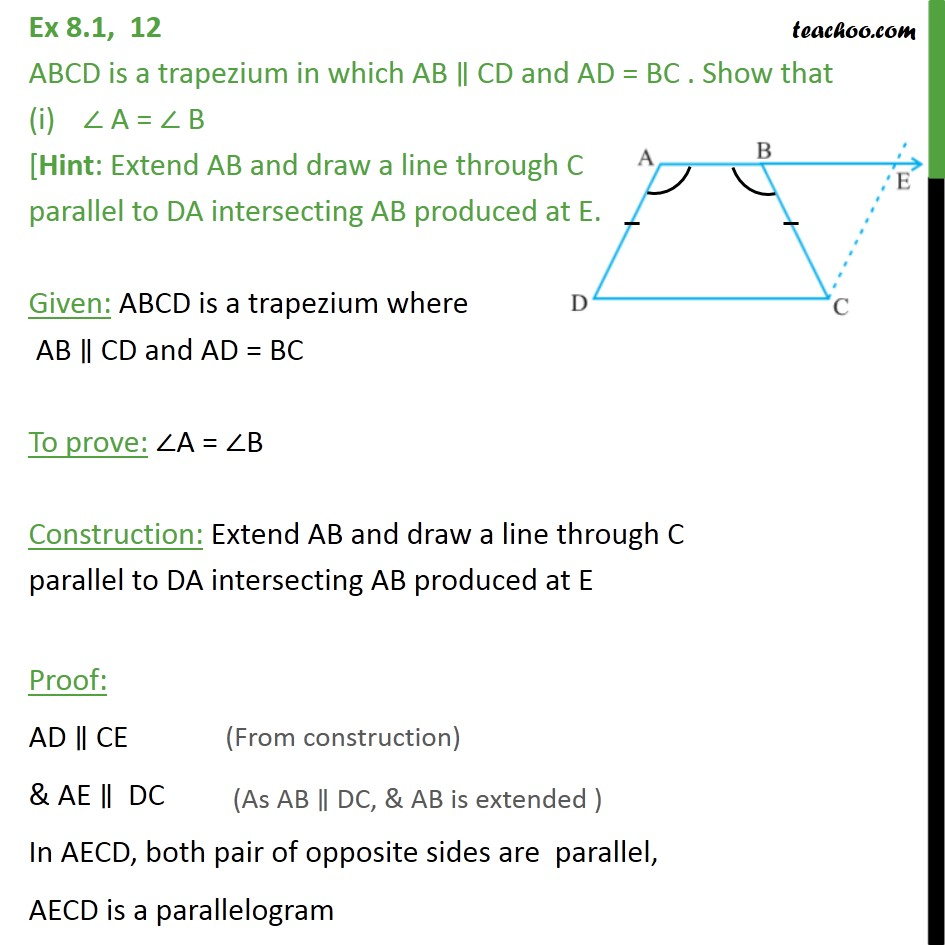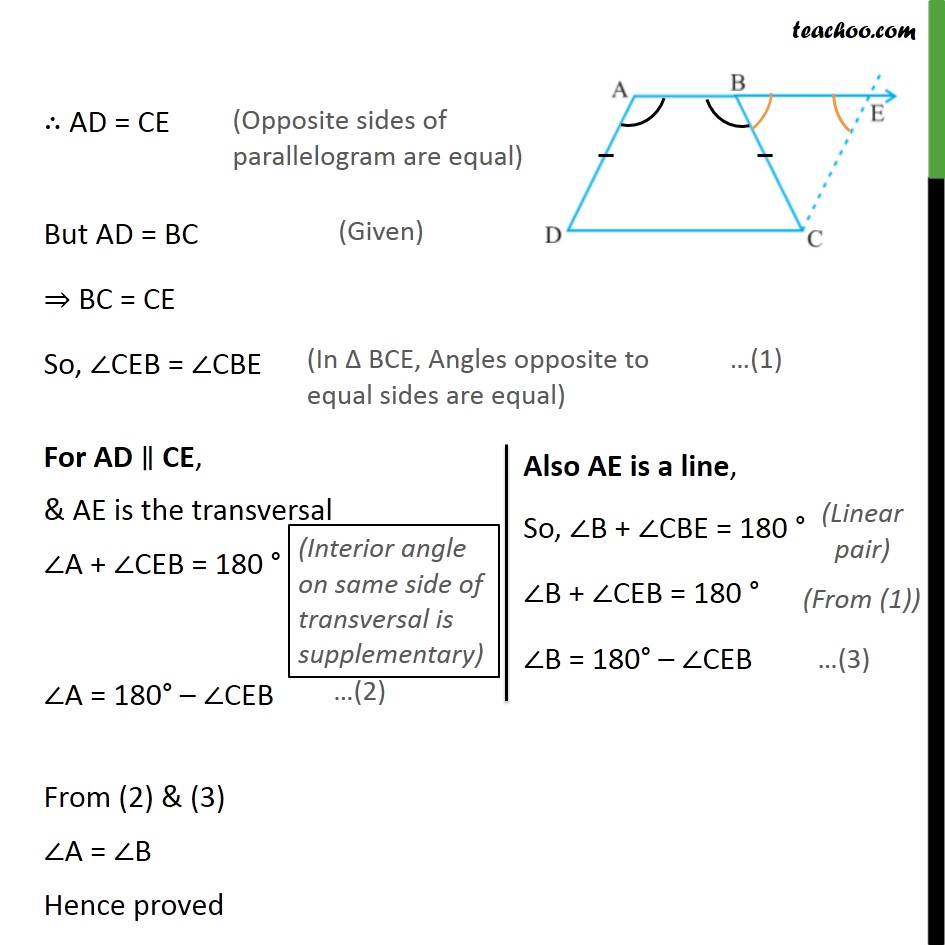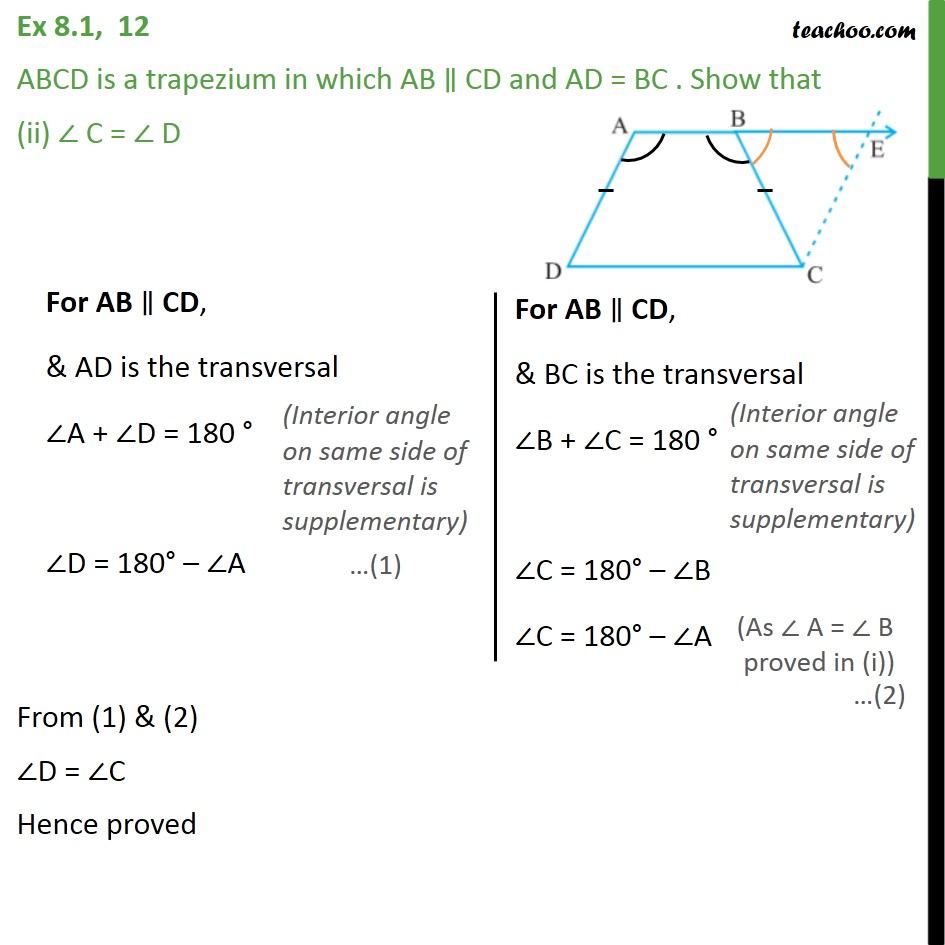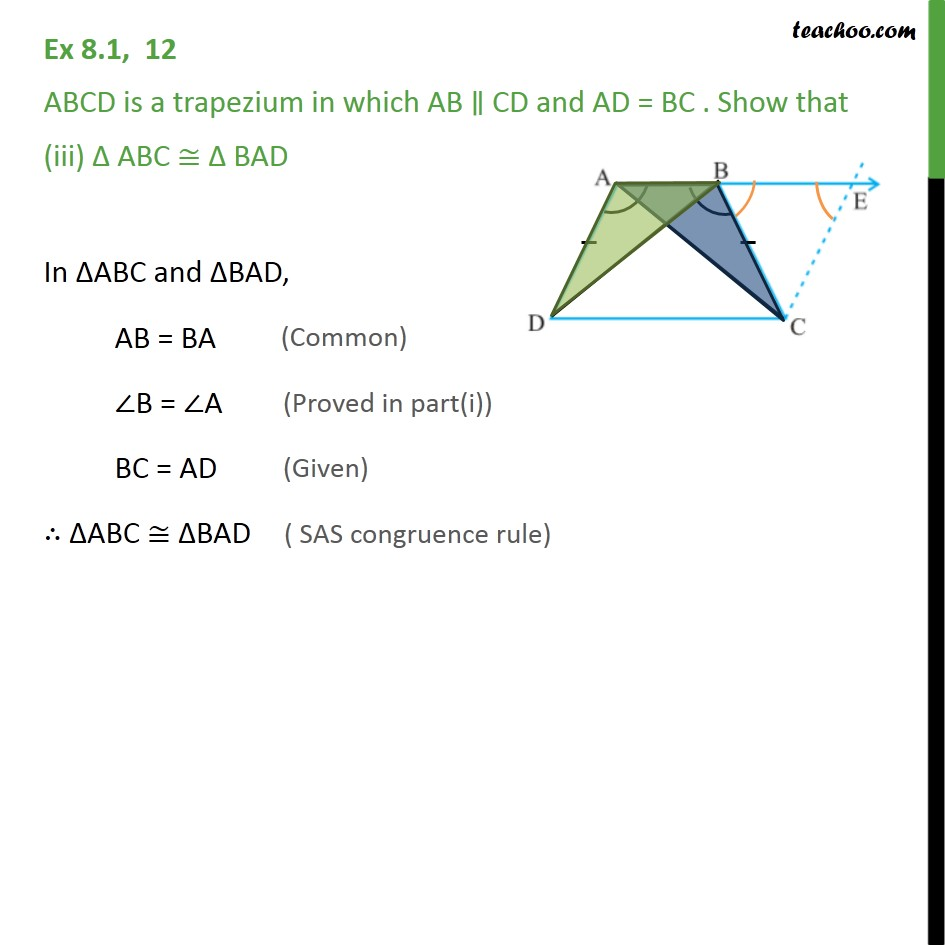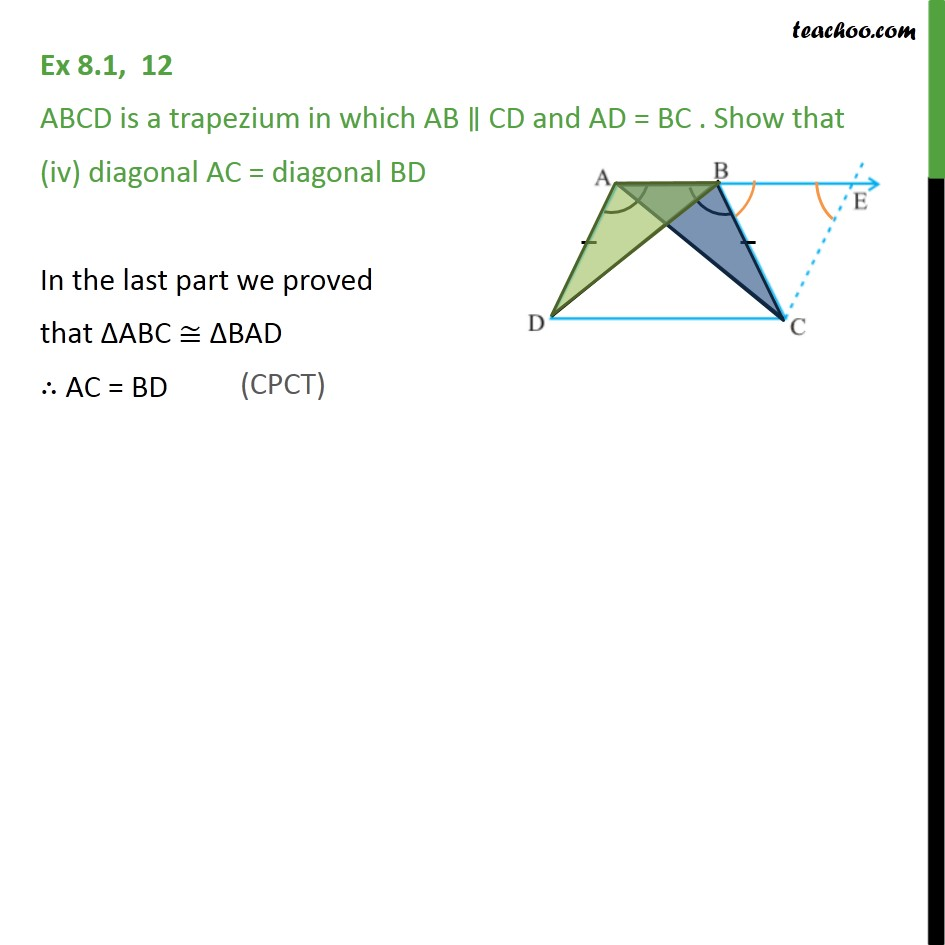1. Chapter 8 Class 9 Quadrilaterals (Term 2)
2. Serial order wise
3. Ex 8.1

Transcript

Ex 8.1, 12 ABCD is a trapezium in which AB CD and AD = BC . Show that A = B [Hint: Extend AB and draw a line through C parallel to DA intersecting AB produced at E. Given: ABCD is a trapezium where AB CD and AD = BC To prove: A = B Construction: Extend AB and draw a line through C parallel to DA intersecting AB produced at E Proof: AD CE & AE DC In AECD, both pair of opposite sides are parallel, AECD is a parallelogram AD = CE But AD = BC BC = CE So, CEB = CBE From (2) & (3) A = B Hence proved Ex 8.1, 12 ABCD is a trapezium in which AB CD and AD = BC . Show that (ii) C = D From (1) & (2) D = C Hence proved Ex 8.1, 12 ABCD is a trapezium in which AB CD and AD = BC . Show that (iii) ABC BAD In ABC and BAD, AB = BA B = A BC = AD ABC BAD Ex 8.1, 12 ABCD is a trapezium in which AB CD and AD = BC . Show that (iv) diagonal AC = diagonal BD In the last part we proved that ABC BAD AC = BD

Ex 8.1